# Optimizing sample sizes in A/B testing, Part III: Aggregate time-discounted expected lift

This is Part III of a three part blog post on how to optimize your sample size in A/B testing. Make sure to read Part I and Part II if you haven't already.

In Part II, we learned how before the experiment starts we can estimate $\hat{L}$, the expected post-experiment lift, a probability weighted average of outcomes.

In Part III, we’ll discuss how to estimate what is perhaps the most important per-unit cost of experimentation: the forfeited benefits that are lost by delayed shipment. This leads to something I think is incredibly cool: A formula for the aggregate time-discounted expected post-experiment lift as a function of sample size. We call this quantity $\hat{L}_a$. The formula for $\hat{L}_a$ allows you to pick optimal sample sizes specific to your business circumstances. We’ll cover two examples in Python, one where you are testing a continuous variable, and one where you are testing a binary variable (as in conversion rate experiments).

As usual, the focus will be on choosing a sample size at the beginning of the experiment and committing to it, not on dynamically updating the sample size as the experiment proceeds.

## A quick modification from Part II

In Part II, we saw that if you ship whichever version (A or B) does best in the experiment, your business will on average experience a post-experiment per-user lift of

where $\sigma_\Delta^2$ is the variance on your normally distributed zero-mean prior for $\mu_B - \mu_A$, $\sigma_X^2$ is the within-group variance, and $n$ is the per-bucket sample size.

Because Part III is primarily concerned with the duration of the experiment, we’re going to modify the formula to be time-dependent. As a simplifying assumption we’re going to make sessions, rather then users, the unit of analysis. We’ll also assume that you have a constant number of sessions per day. This changes the definition of $\hat{L}$ to a post-experiment per-session lift, and the formula becomes

where $m$ is the sessions per day for each bucket, and $\tau$ is duration of the experiment in days.

## Time Discounting

The formula above shows that larger sample sizes result in higher $\hat{L}$, since larger samples make it more likely you will ship the better version. But as with all things in life, there are costs to increasing your sample size. In particular, the larger your sample size, the longer you have to wait to ship the winning bucket. This is bad because lift today is much more valuable than the same lift a year from now.

How much more valuable is lift today versus lift a year from now? A common way to quantify this is with exponential discounting, such that weights (or “discount factors”) on future lift follow the form:

where $r$ is a discount rate. For startup teams, the annual discount rate might be quite large, like 0.5 or even 1.0, which would correspond to a daily discount rate $r$ of 0.5/365 or 1.0/365, respectively. Figure 1 shows an example of a discount rate of 1.0/365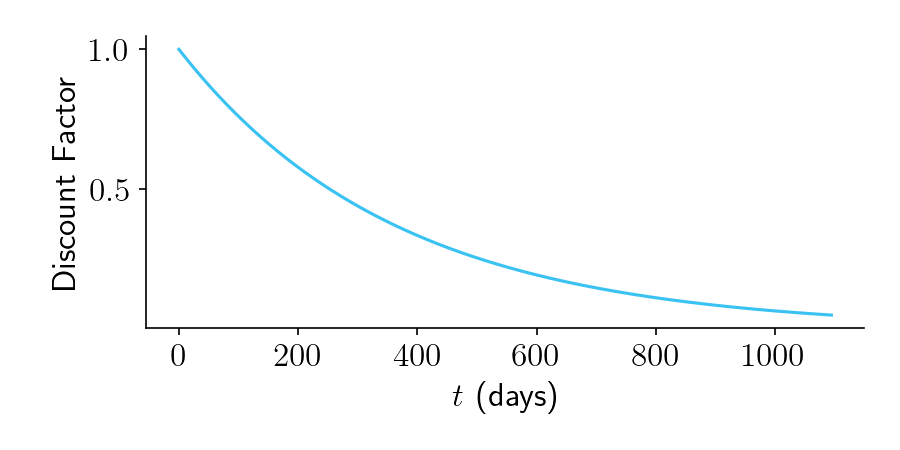Figure 1.

## Aggregate time-discounted expected lift: Visual Intuition

Take a look at Figure 2, below. It shows an experiment that is planned to run for $\tau = 60$ days. The top panel shows $\hat{L}$, which we have now defined as the expected per-session lift. While the experiment is running, $\hat{L} = 0$, since our prior is that $\Delta$ is sampled from a normal distribution with mean zero. But once the experiment finishes and we launch the winning bucket, we should begin to reap our expected per-session lift.

The middle panel shows our discount function.

The bottom panel shows our time-discounted lift, defined as the product of the lift in the top panel and the time discount in the middle panel. (We can also multiply it by $M$, the number of post-experiment sessions per day, which for simplicity we set to 1 here.) The aggregate time-discounted expected lift, $\hat{L}_a$, is the area under the curve.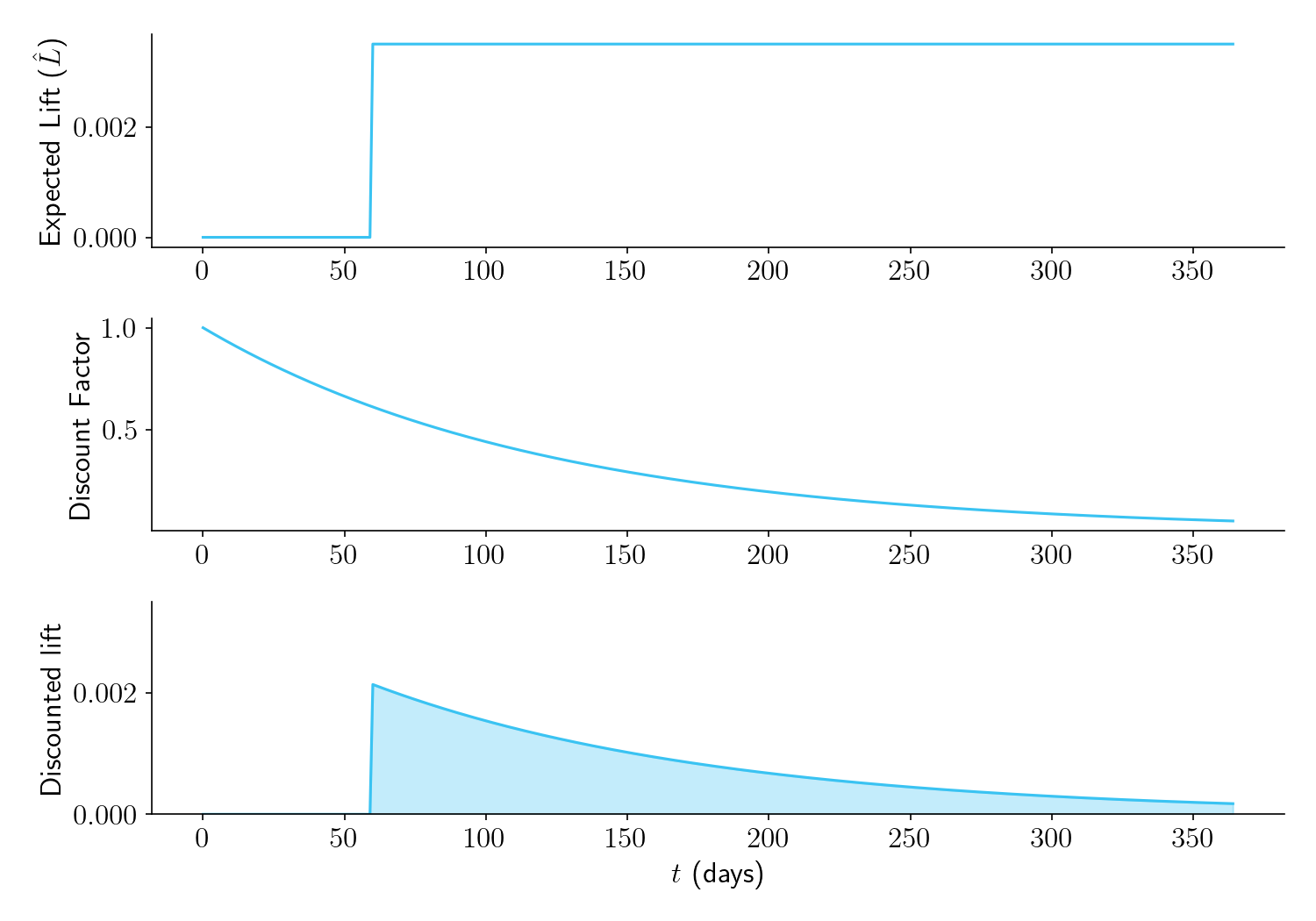Figure 2.

Now let’s see what happens with different experiment durations. Figure 3 shows that the longer you plan to run your experiment, the higher $\hat{L}$ will be (top panel). But due to time discounting, (middle panel), the area under the time-discounted lift curve (bottom panel) is low for overly large sample sizes. There is an optimal duration of the experiment (in this case, $\tau = 24$ days), that maximizes $\hat{L}_a$, the area under the curve.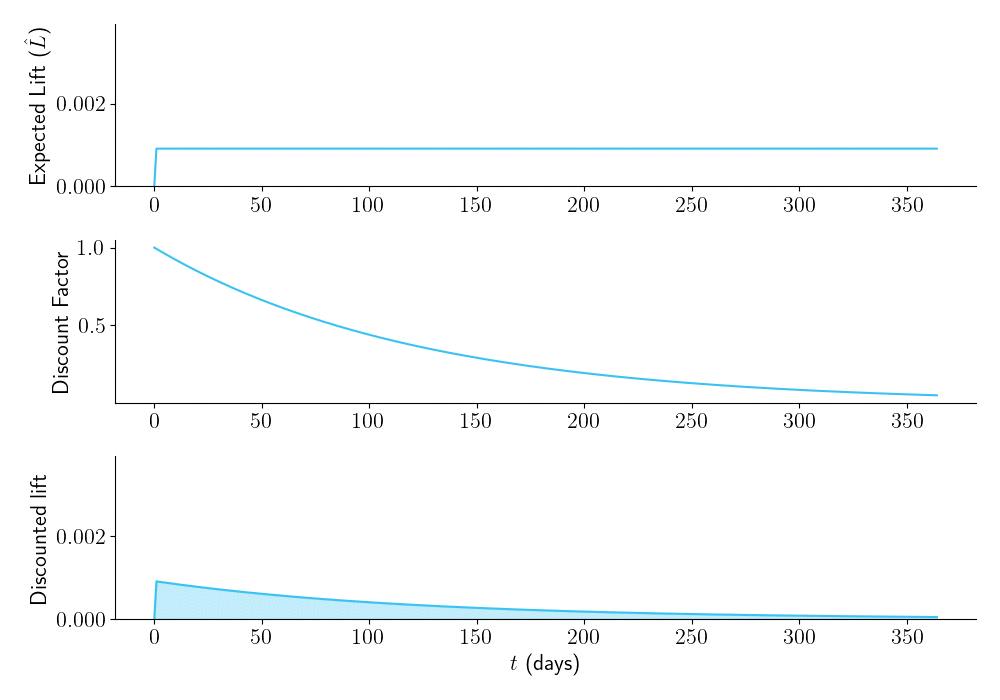Figure 3.

## Aggregate time-discounted expected lift: Formula

The aggregate time-discounted expected lift $\hat{L}_a$, i.e. the area under the curve in the bottom panel of Figure 3, is:

where $\tau$ is the duration of the experiment and $M$ is the number of post-experiment sessions per day. See the Appendix for a derivation.

1. Increasing the number of bucketed sessions per day, $m$, always increases $\hat{L}_a$.
2. Increasing the duration of the experiment, $\tau$, may or may not help. It’s impact is controlled by competing forces in the numerator and denominator. In the numerator, higher $\tau$ decreases $\hat{L}_a$ by delaying shipment. In the denominator, higher $\tau$ increases $\hat{L}_a$ by making it more likely you will ship the superior version.

## Optimizing sample size

At long last, we can answer the question, “How long should we run this experiment?”. A nice way to do it is to plot $\hat{L}_a$ as a function of $\tau$. Below we see what this looks like for one set of parameters. Here the optimal duration is 38 days.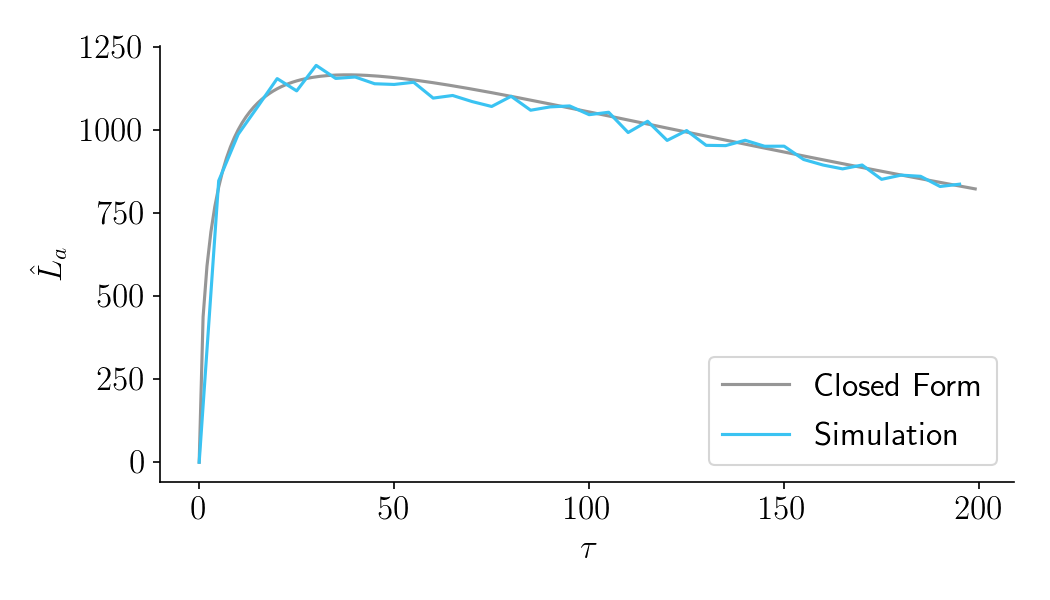Figure 4.

Note also that a set of simulated experiment and post-experiment periods (in blue) confirm the predictions of the closed form solution (in gray). See the notebook for details.

## Examples in Python

### Example 1: Continuous variable metric

Let’s say you want to run an experiment comparing two different versions of a website, and your main metric is revenue per session. You know in advance that the within-group variance of this metric is $\sigma_X^2 = 100$. You don’t know which version is better but you have a prior that the true difference in means is normally distributed with variance $\sigma_\Delta^2 = 1$. You have 200 sessions per day and plan to bucket 100 sessions into Version A and 100 sessions into Version B, running the experiment for $\tau=20$ days. Your discount rate is fairly aggressive at 1.0 annually, or $r = 1/365$ per day. Using the function in the notebook, you can find $\hat{L}_a$ with this command:

get_agg_lift_via_closed_form(var_D=1, var_X=100, m=100, tau=25, r=1/365, M=200)
# returns 26298


You can also use the find_optimal_tau function to determine the optimal duration, which in this case is $\tau=18$.

### Example 2: Conversion rates

Let’s say your main metric is conversion rate. You think that on average conversion rates will be about 10%, and that the difference in conversion rates between buckets will be normally distributed with variance 1%. Using the normal approximation of the binomial distribution, you can use p*(1-p) for var_X.

p = 0.1
get_agg_lift_via_closed_form(var_D=0.01**2, var_X=p*(1-p), m=100, tau=25, r=1/365, M=200)
# returns 207


You can also use the find_optimal_tau function to determine the optimal duration, which in this case is $\tau=49$.

## FAQ

Q: Has there been any similar work on this?

A: As I was writing this, I came across a fantastic in-press paper by Elea Feit and Ron Berman. The paper is exceptionally clear and I would recommend reading it. Like this blog post, Feit and Berman argue that it doesn’t make any sense to pick sample sizes based on statistical significance and power thresholds. Instead they recommend profit-maximizing sample sizes. They independently come to the same formula for $\hat{L}$ as I do (see right addend in their Equation 9, making sure to substitute my $\frac{\sigma_\Delta^2}{2}$ for their $\sigma^2)$. Where they differ is that they assume there is a fixed pool of $N$ users that can only experience the product once. In their setup, you can allocate $n_1$ users to Bucket A and $n_2$ users to Bucket B. Once you have identified the winning bucket, you ship that version to the remaining $N-n_1-n_2$ users. Your expected profit is determined by the total expected lift from those users. My experience in industry differs from this setup. In my experience there is no constraint that you can only show the product once to a fixed set of users. Instead, there is often an indefinitely increasing pool of new users, and once you ship the winning bucket you can ship it to everyone, including users who already participated in the experiment. To me, the main constraint in industry is therefore time discounting, rather than a finite pool of users.

Q: In addition to the lift from shipping a winning bucket, doesn’t experimentation also help inform us about the types of products that might work in the future? And if so, doesn’t this mean we should run experiments longer than recommended by your formula for $\hat{L}_a$?

A: Yes, experimentation can teach lessons that are generalizable beyond the particular product being tested. This is an advantage of high powered experimentation not included in my framework.

Q: What about novelty effects? Yup, that’s a real concern not covered by my framework. You probably want to know a somewhat long term impact of your product, which means you should probably run the experiment for longer than recommended by my framework.

Q: If some users can show up in multiple sessions, doesn’t bucketing by session violate independence assumptions?

A: Yeah, so this is tricky. For many companies, there is a distribution of user activity, where some users come for many sessions per week and other users come for only one session at most. Modeling this would make the framework significantly more complicated, so I tried to simplify things by making sessions the unit of analysis.

Q: Is there anything else on your blog vaguely related to this topic?

## Appendix

The aggregate time-discounted expected lift $\hat{L}_a$ is

where $\hat{L}$ is the expected per-session lift, $M$ is the number of post-experiment sessions per day, $r$ is the discount rate, and $\tau$ is the duration of the experiment. Solving the integral gives:

Plugging in our previously solved value of $\hat{L}$ gives

# Optimizing sample sizes in A/B testing, Part II: Expected lift

This is Part II of a three-part blog post on how to optimize your sample size in A/B testing. Make sure to read Part I if you haven't already.

In this blog post (Part II), I describe what I think is an incredibly cool business-focused formula that quantifies how much you can benefit from increasing your sample size. It is, in short, an average of the value of all possible outcomes of the experiment, weighted by their probabilities. This post starts off kind of dry, but if you can make it through the first section, it gets a lot easier.

## Outcome probabilities

Imagine you are comparing two versions of a website. You currently are on version A, but you would like to compare it to version B. Imagine you are measuring some random variable $X$, which might represent something like clicks per user or page views per user. The goal of the experiment is to determine which version of the website has a higher mean value of $X$.

This blog post aims to quantify the benefit of experimentation as an average of the value of all possible outcomes, weighted by their probabilities. To do that, we first need to describe the probabilities of all the different outcomes. An outcome consists of two parts: A true difference in means, $\Delta$, defined as

and an experimentally observed difference in means $\delta$, defined as

Let’s start with $\Delta$. While you don’t yet know which version of the website is better (that’s what the experiment is for!), you have a sense for how important the product change is. You can therefore create a normally distributed prior on $\Delta$ with mean zero and variance $\sigma_\Delta^2$.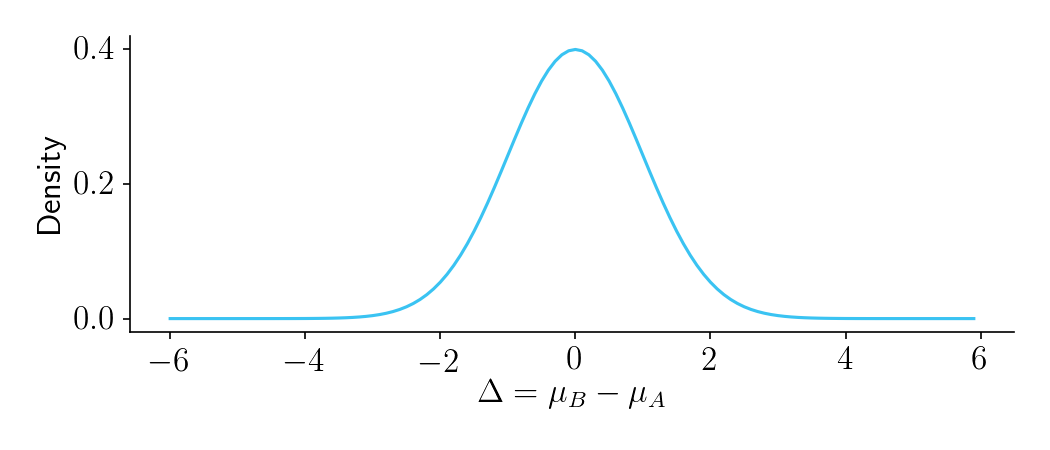Figure 1.

Next, let’s consider $\delta$, your experimentally observed difference in means. It will be a noisy estimate of $\Delta$. Let’s assume you have previously measured the variance of $X$ to be $\sigma_X^2$. It is reasonable to assume that within each group in the experiment, and for any particular $\Delta$, the variance of $X$ will still be $\sigma_X^2$. You should therefore believe that for any particular $\Delta$, the observed difference in means $\delta$ will be sampled from a normal distribution $\mathcal{N}(\Delta, \sigma_c^2)$, where

and where $n$ is the sample size in each of the two buckets. If that doesn’t make sense, check out this video.

Collectively, this all forms a bivariate normal distribution of outcomes, shown below.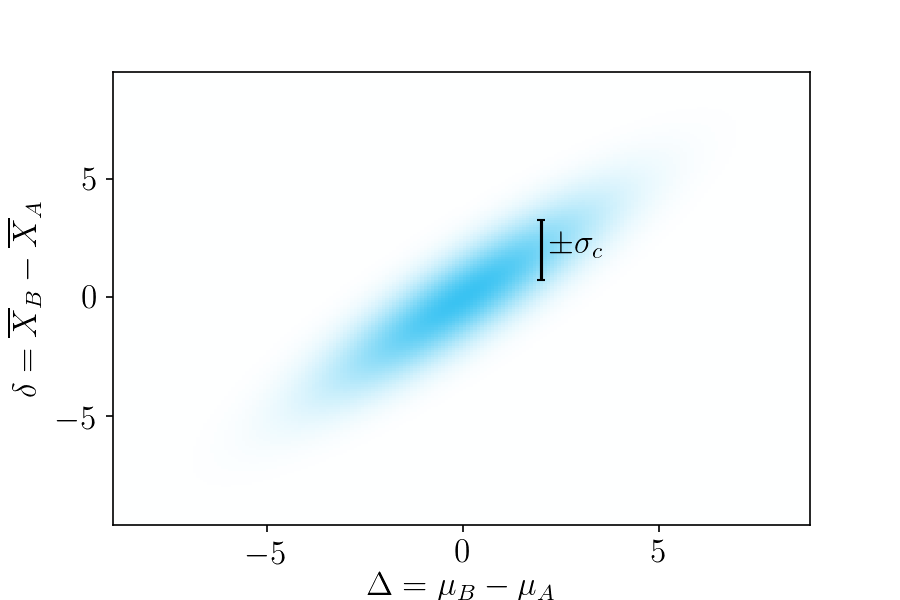Figure 2. Probabilities of possible outcomes, based on your prior beliefs. The horizontal axis is the true difference in means, and the vertical axis is the observed difference in means.

To gain some more intuition about this, take a look at Figure 3. As sample size increases, $\sigma^2_c$ decreases.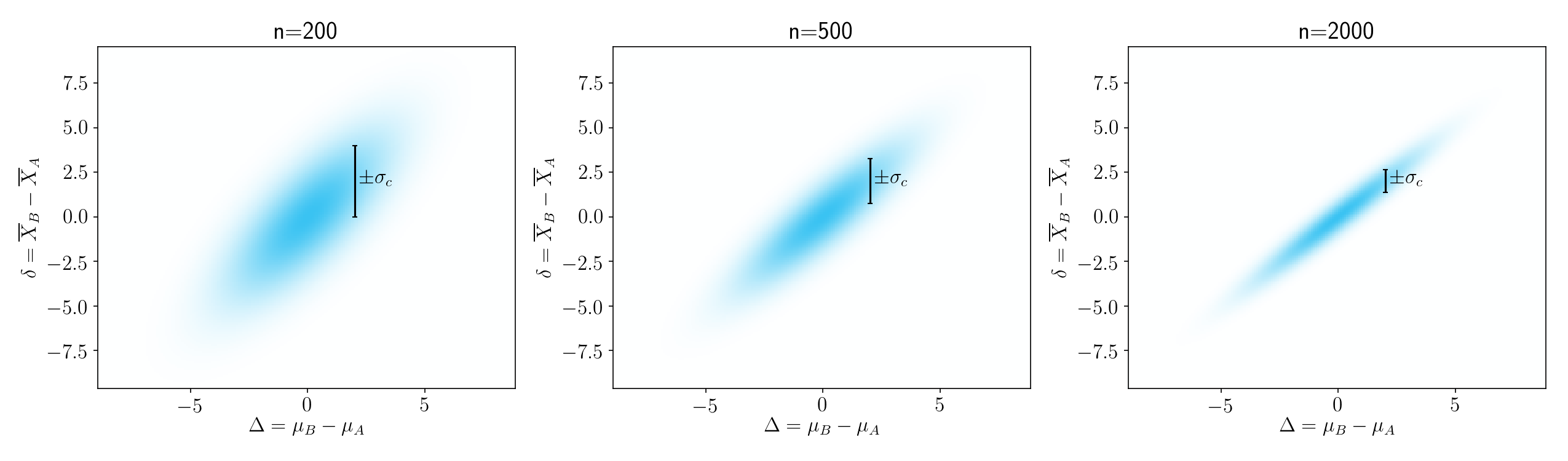Figure 3.

## Outcome lifts

Now that we know the probabilities of all the different outcomes, we next need to estimate how much per-user lift, $l$, we will gain from each possible outcome, assuming we follow a policy of shipping whichever bucket (A or B) looked better in the experiment.

• In cases where $\delta > 0$ and $\Delta > 0$, you would ship B and your post-experiment per-user lift will be positively valued at $l = \Delta$.
• In cases where $\delta > 0$ and $\Delta < 0$, you would ship B, but unfortunately your post-experiment per-user lift will be negatively valued at $l = \Delta$, since $\Delta$ is negative.
• In cases where $\delta < 0$, you would keep A in production, and your post-experiment lift would be zero.

A heatmap of the per-user lifts ($l$) for each outcome is shown in the plot below. Good outcomes, where shipping B was the right decision, are shown in blue. Bad outcomes, where shipping B was the wrong decision, are shown in red. There are two main ways to get a neutral outcomes, shown in white. Either you keep A (bottom segment), in which case there is zero lift, or you ship B where B is only negligibly different than A (vertical white stripe).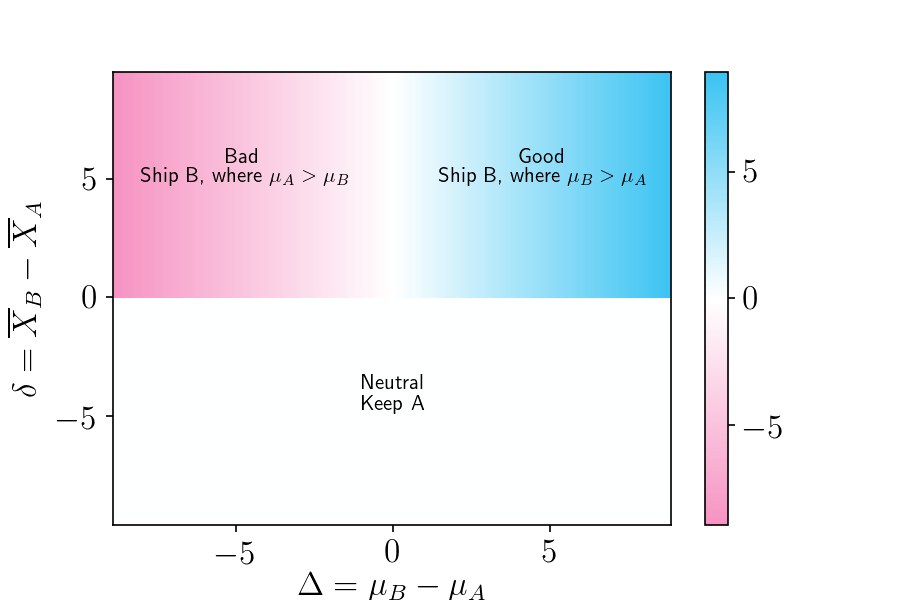Figure 4. Heatmap of possible outcomes, where the color scale represents the lift, $l$. The horizontal axis is the true difference in means, and the vertical axis is the observed difference in means

## Probability-weighted Outcome Lifts

At this point, we know the probability of each outcome, and we know the post-experiment per-user lift of each outcome. To determine how much lift we can expect, on average, by shipping the winning bucket of an experiment, we need to compute a probability-weighted average of the outcome lifts. Let’s start by looking at this visually and then later we’ll get into the math.

As shown in Figure 5, if we multiply the bivariate normal distribution (left) by the lift map (center), we can obtain the probability-weighted lift of each outcome (right).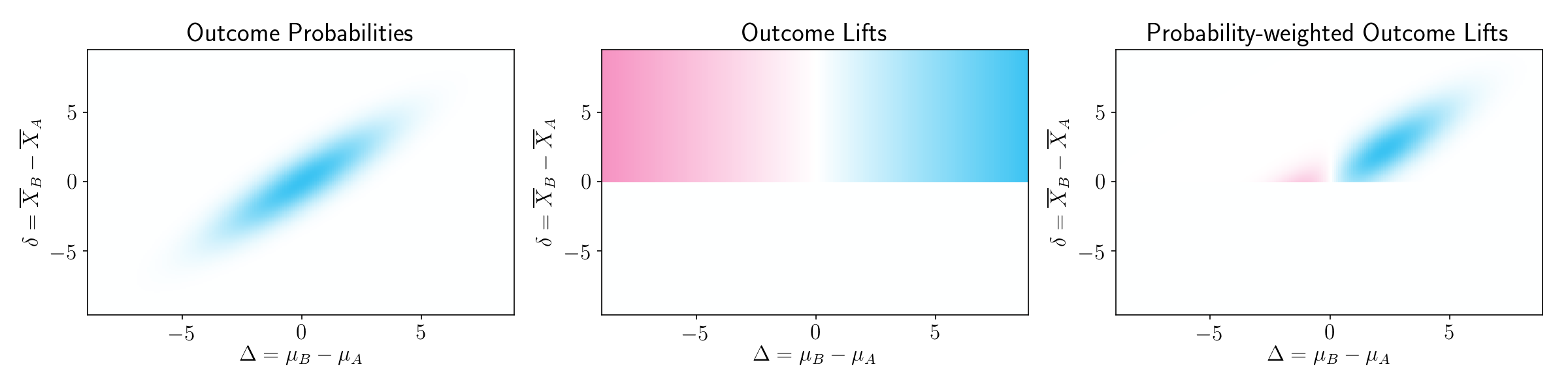Figure 5.

The good outcomes contribute more than the bad outcomes, simply because a good outcome is more likely than a bad outcome. To put it differently, experimentation will on average give you useful information.

To gain some more intuition on this, it is helpful to see this plot for different sample sizes. As sample size increases, the probability-weighted contribution of bad outcomes gets smaller and smaller.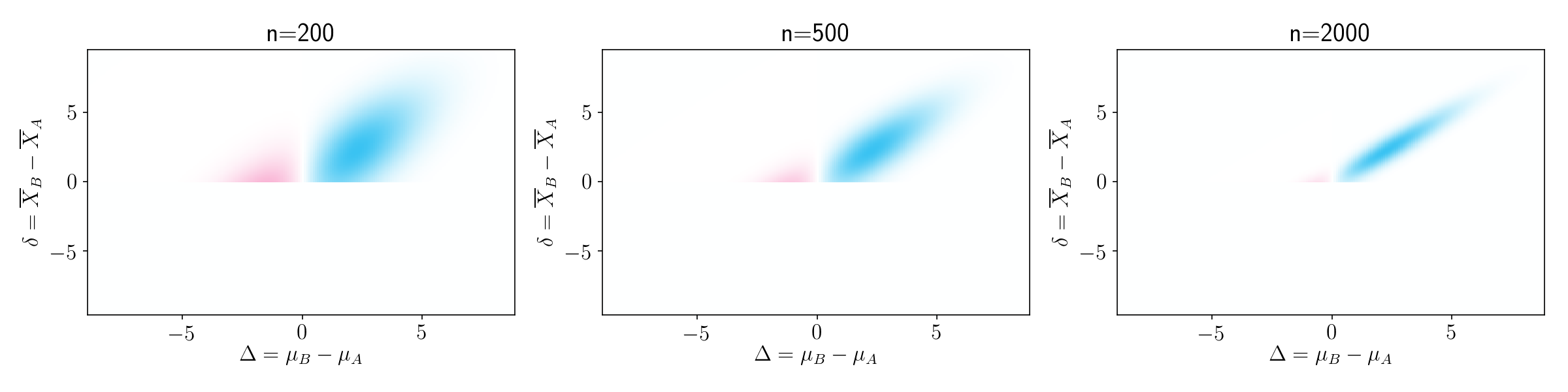Figure 6.

### Computing the expected post-experiment per-user lift

We’re almost there! To determine the expected post-experiment lift from shipping the winning bucket, we need to compute a probability-weighted average of all the post-experiment lifts. In other words, we need to sum up all the probability-weighted post-experiment lifts on the right panel of Figure 5. The formula for doing this is shown below. A derivation can be found in the Appendix.

• As $n$ increases, $\hat{L}$ increases. This makes sense. The larger the sample size, the more likely it is that you’ll ship the winning bucket.
• As the within-group variance $\sigma_X^2$ increases, $\hat{L}$ decreases. That’s because a high within-group variance makes experiments less informative – they’re more likely to give you the wrong answer.
• As the variance prior on $\Delta$ increases, $\hat{L}$ increases. This also make sense. The more impactful (positive or negative) you think the product change might be, the more value you will get from experimentation.

You can try this out using the get_lift_via_closed_form formula in the Notebook.

## Demonstration via simulation

In the previous section, we derived a formula for $\hat{L}$. Should you trust a formula you found on a random internet blog? Yes! Let’s put the formula to the test, by comparing its predictions to actual simulations.

First, let’s consider the case where the outcome is a continuous variable, such as the number of clicks. Let’s set $\sigma_D^2 = 2$ and $\sigma_X^2 = 100$. We then measure $\hat{L}$ for a range of sample sizes, using both the closed-form solution and simulations. To see how we determine $\hat{L}$ for simulations, refer to the box below.

Procedure for finding $\hat{L}$ with simulations

Loop through thousands of simulated experiments. On each each experiment doing the following:

1. Sample a true group difference $\Delta$ from $\mathcal{N}(0, \sigma_D^2)$
2. Sample an $X$ for each of the $n$ users in each bucket A and B, using Normal distributions $\mathcal{N}(\frac{\Delta}{2}, \sigma_X^2)$ and $\mathcal{N}(-\frac{\Delta}{2}, \sigma_X^2)$, respectively.
3. Compute $\delta = \overline{X}_B - \overline{X}_A$.
4. If $\delta <= 0$, stick with A and accrue zero lift.
5. If $\delta > 0$, ship B and accrue the per-user lift of $\Delta$, which will probably, but not necessarily, be positive.
We run these experiments thousands of times, each time computing the per-user lift. Finally, we average all the per-user lifts together to get $\hat{L}$. See the get_lift_via_simulations_continuous function in the notebook for an implementation.

As seen in Figure 7, below, the results of the simulation closely match the closed-form solution.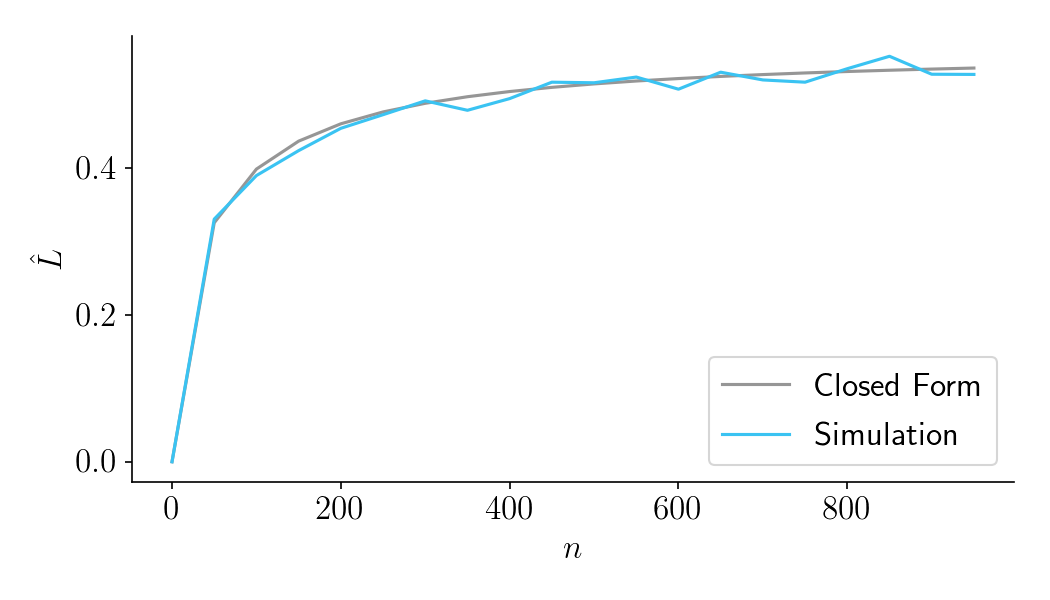Figure 7.

Second, let’s consider the case where the variable is binary, as in conversion rates. For reasonably large values of $n$, we can safely assume that the error variance is normally distributed with variance $\sigma_X^2 = p(1-p)$, where $p$ is the baseline conversion rate. For this example, let’s set the baseline conversion rate $p = 0.1$, and let’s set $\sigma_\Delta^2 = 0.01^2$. The results of the simulation closely match the closed-form solution.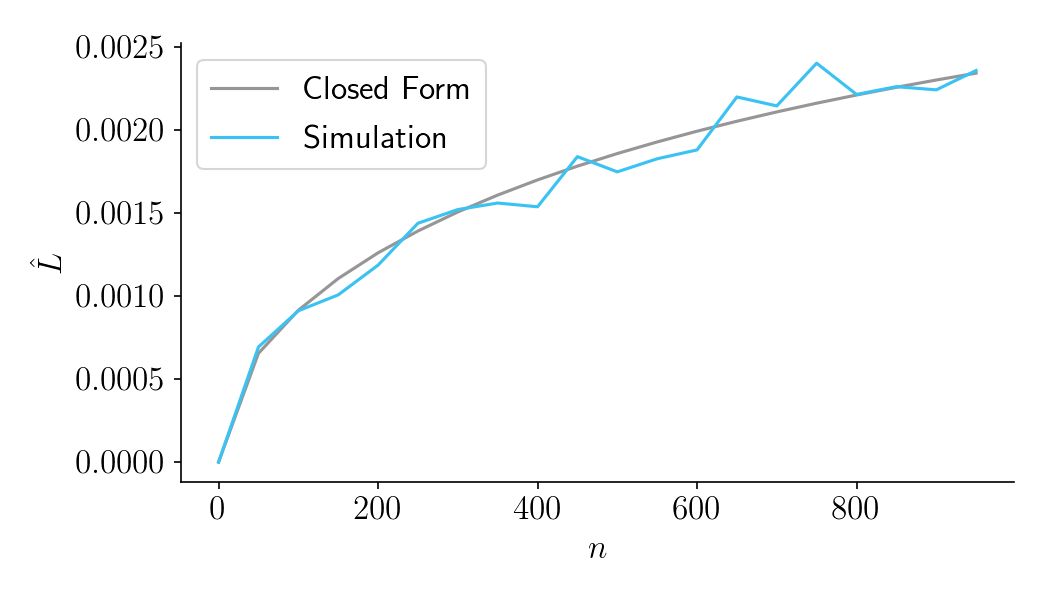Figure 8.

## Thinking about costs, and a preview of Part III

In this blog post, we saw how increasing the sample size improves the expected post-experiment per-user lift, $\hat{L}$. But to determine the optimal sample size, we need to think about costs.

The cost in dollars of an experiment can be described as $f + vn$, where $f$ is a fixed cost and $v$ is the variable cost per participant. If you already know these costs, and if you already know the revenue increase $u$ from each unit increase in lift, you can calculate the net revenue $R$ as

and then find the sample size $n$ that maximizes $R$.

Unfortunately, these costs aren’t always readily available. The good news is that there is a really nice way to calculate the most important cost: the forfeited benefit that comes from prolonging your experiment. To read about that, and about how to optimize your sample size, please continue to Part III.

## Appendix

To determine $\hat{L}$, we start with the probability-weighted lifts on the right panel of Figure 5. This is a bivariate normal distribution over $\Delta$ and $\delta$, multiplied by $\Delta$.

where the correlation coefficient $\rho$, is defined as:

and $\sigma_\delta^2$ is the variance on $\delta$. By the variance addition rules, $\sigma_\delta^2$ is defined as

We next need to sum up the probability-weighted values in $f(\Delta, \delta)$. To obtain a closed form solution, we can use integration.

The integration limits on $\delta$ start at zero because the lift will always be zero if $\delta < 0$ (i.e if the status quo bucket A wins the experiment).

Thanks to my 15-day free trial of Mathematica, I determined that this integral comes out to the surprisingly simple

The command I used was:

Integrate[(t / (2*\[Pi]*s1*s2*Sqrt[1 - p^2]))*Exp[-((t^2/s1^2 - \
(2*p*t*d)/(s1*s2) + d^2/s2^2)/(2*(1 - p^2)))], {d, 0, \[Infinity]}, \
{t, -\[Infinity], \[Infinity]}, Assumptions -> p > 0 && p < 1 && s1 > \
0 && s2 > 0]


If we then substitute in previously defined formulas for $\rho$ and $\sigma_c^2$, we can produce a formula that accepts more readily-available inputs.

Continue to Part III.

# Optimizing sample sizes in A/B testing, Part I: General summary

A special thanks to John McDonnell, who came up with the idea for this post. Thanks also to Marika Inhoff and Nelson Ray for comments on an earlier draft.

If you’re a data scientist, you’ve surely encountered the question, “How big should this A/B test be?”

The standard answer is to do a power analysis, typically aiming for 80% power at $\alpha$=5%. But if you think about it, this advice is pretty weird. Why is 80% power the best choice for your business? And doesn’t a 5% significance cutoff seem pretty arbitrary?

In most business decisions, you want to choose a policy that maximizes your benefits minus your costs. In experimentation, the benefit comes from learning information to drive future decisions, and the cost comes from the experiment itself. The optimal sample size will therefore depend on the unique circumstances of your business, not on arbitrary statistical significance thresholds.

In this three-part blog post, I’ll present a new way of determining optimal sample sizes that completely abandons the notion of statistical significance.

• Part I: General Overview. Starts with a mostly non-technical overview and ends with a section called “Three lessons for practitioners”.
• Part II: Expected lift. A more technical section that quantifies the benefits of experimentation as a function of sample size.
• Part III: Aggregate time-discounted lift. A more technical section that quantifies the costs of experimentation as a function of sample size. It then combines costs and benefits into a closed-form expression that can be optimized. Ends with an FAQ.

Throughout Parts I-III, the focus will be on choosing a sample size at the beginning of the experiment and committing to it, not on dynamically updating the sample size as the experiment proceeds.

With that out of the way, let’s get started!

### Benefits of large samples

The bigger your sample size, the more likely it is that you’ll ship the right bucket. Since there is a gain to shipping the right bucket and a loss to shipping the wrong bucket, the averages benefit of the experiment is a probability-weighted average of these outcomes. We call this the expected post-experiment lift, $\hat{L}$, which we cover in more detail in Part II.

### Costs of large samples

For most businesses, increasing your sample size requires you to run your experiment longer. This brings us to the main per-unit cost of experimentation: the forfeited benefits that could come from shipping the winning bucket earlier. In a fast moving startup, there’s often good reason to accrue your wins as soon as possible. The advantage of shipping earlier can be quantified with a discount rate, which describes how much you value the near future over the distant future. If you have a high discount rate, it’s critical to ship as soon as possible. If you have a low discount rate, you can afford to wait longer. This is described in more detail in Part III.

### Combining costs and benefits into an optimization function

You should run your experiment long enough that you’ll likely ship the winning bucket, but not so long that you waste time not having shipped your product. The optimal duration depends on the unique circumstances of your business. The overall benefit of running an experiment, as a function of duration and other parameters, is defined as the aggregate time-discounted expected post-experiment lift, or $\hat{L}_a$.

Figure 1 shows $\hat{L}_a$ as a function of experiment duration in days ($\tau$) for one particular set of business parameters. The gray curve shows the result of a closed form solution presented in Part III. The blue curve shows the results of simulated experiments. As you can see, the optimal duration for this experiment should be about 38 days. Simulations match the closed-form predictions.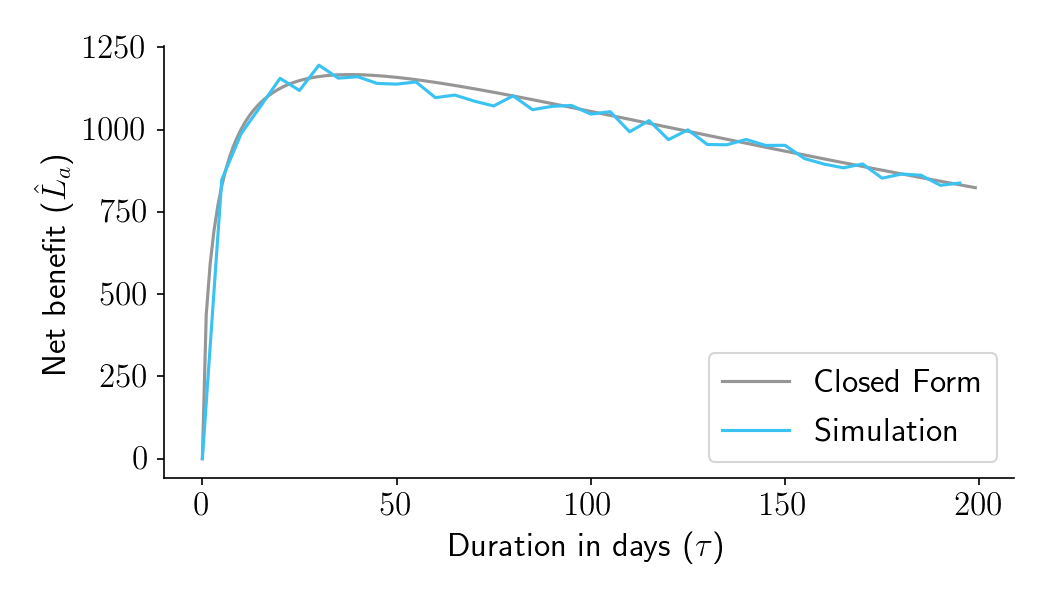Figure 1. Aggregate time-discounted expected post-experiment lift ($\hat{L}_a$) as a function of experiment duration in days ($\tau$), for a fairly typical set of business parameters.

### Three lessons for practitioners

I played around with the formula for $\hat{L}_a$ and came across three lessons that should be of interest to practitioners.

#### 1. You should run “underpowered” experiments if you have a very high discount rate

Take a look at Figure 2, which shows some recommendations for a fairly typical two-bucket conversion rate experiment with 1000 sessions per bucket per day. On the left panel we plot the optimal duration as a function of the annual discount rate. If you have a high discount rate, you care a lot more about the near future than the distant future. It is therefore critical that you ship any potentially winning version as soon as possible. In this scenario, the optimal duration is low (left panel). Power, the probability you will find a statistically significant result, is also low (right panel). For many of these cases, the optimal duration would traditionally be considered “underpowered”.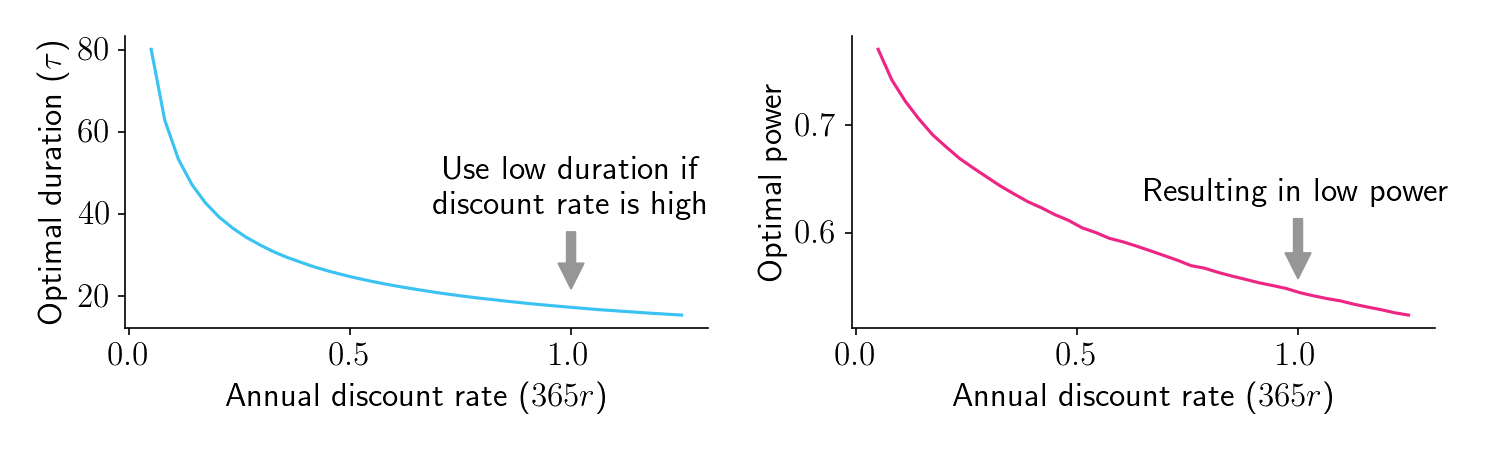Figure 2.

#### 2. You should run “underpowered” experiments if you have a small user base

Now let’s plot these curves as a function of $m$, our daily sessions per bucket. If you only have a small number of daily sessions to work with, you’ll need to run the experiment for longer (left panel). So far, that’s not surprising. But here’s where it gets interesting: Even though optimal duration increases as $m$ decreases, it doesn’t increase fast enough to maintain constant power (right panel). In fact, for low $m$ scenarios where you don’t have a lot of users, the optimal duration results in power that can drop below 50%, well into what would traditionally be considered “underpowered” territory. In these situations, waiting to get a large number of sessions causes too much time-discounting loss.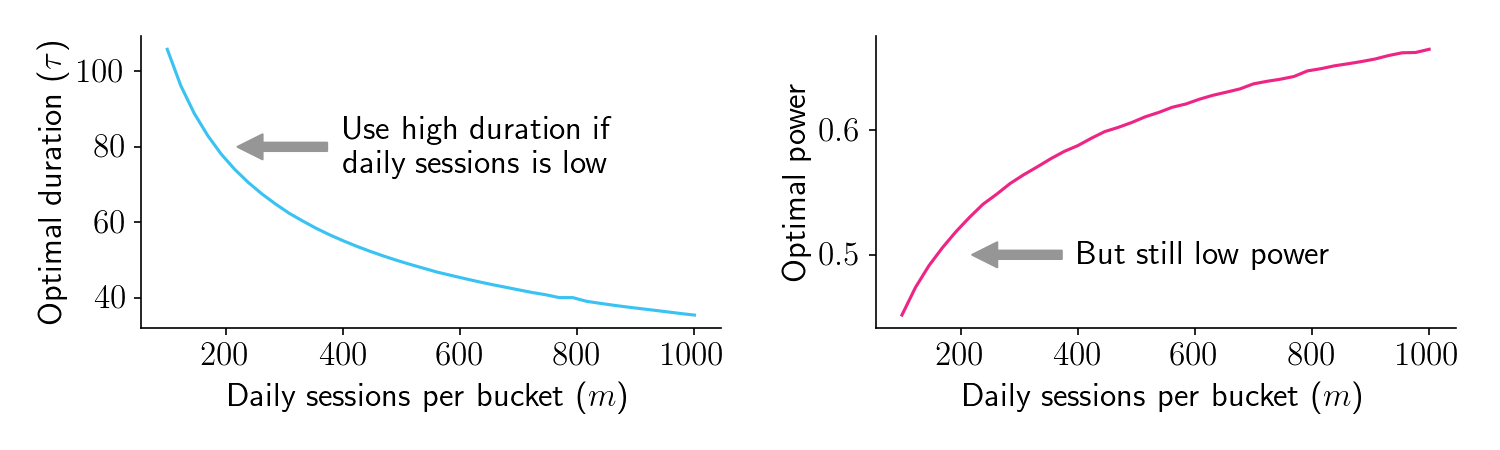Figure 3.

#### 3. That said, it’s far better to run your experiment too long than too short

Let’s take another look at $\hat{L}_a$ as a function of duration. As shown in Figure 4 below, the left shoulder is steeper than the right shoulder. This means that it’s really bad if your experiment is shorter than optimal, but it’s kind of ok if your experiment is longer than optimal.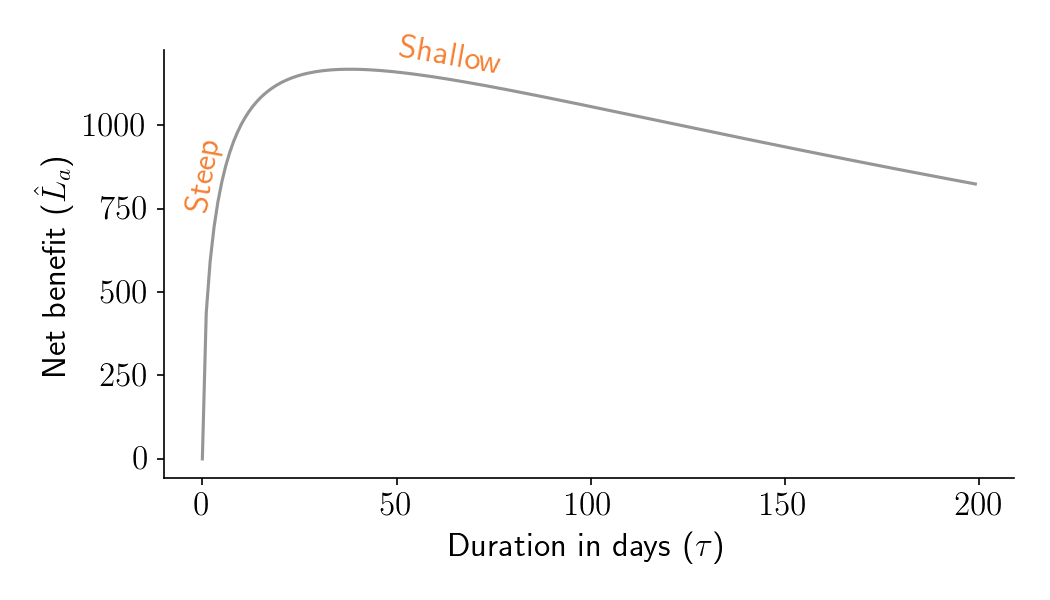Figure 4. Aggregate time-discounted expected post-experiment lift ($\hat{L}_a$) as a function of experiment duration in days ($\tau$), for a fairly typical set of business parameters.

Is this true in general? Yes. Below we plot $\hat{L}_a$ as a function of duration for various combinations of $m$ and the discount rate, $r$. For all of these parameter combinations, it’s better to run a bit longer than optimal than a bit shorter than optimal. The only exception is if you have an insanely high discount rate (not shown).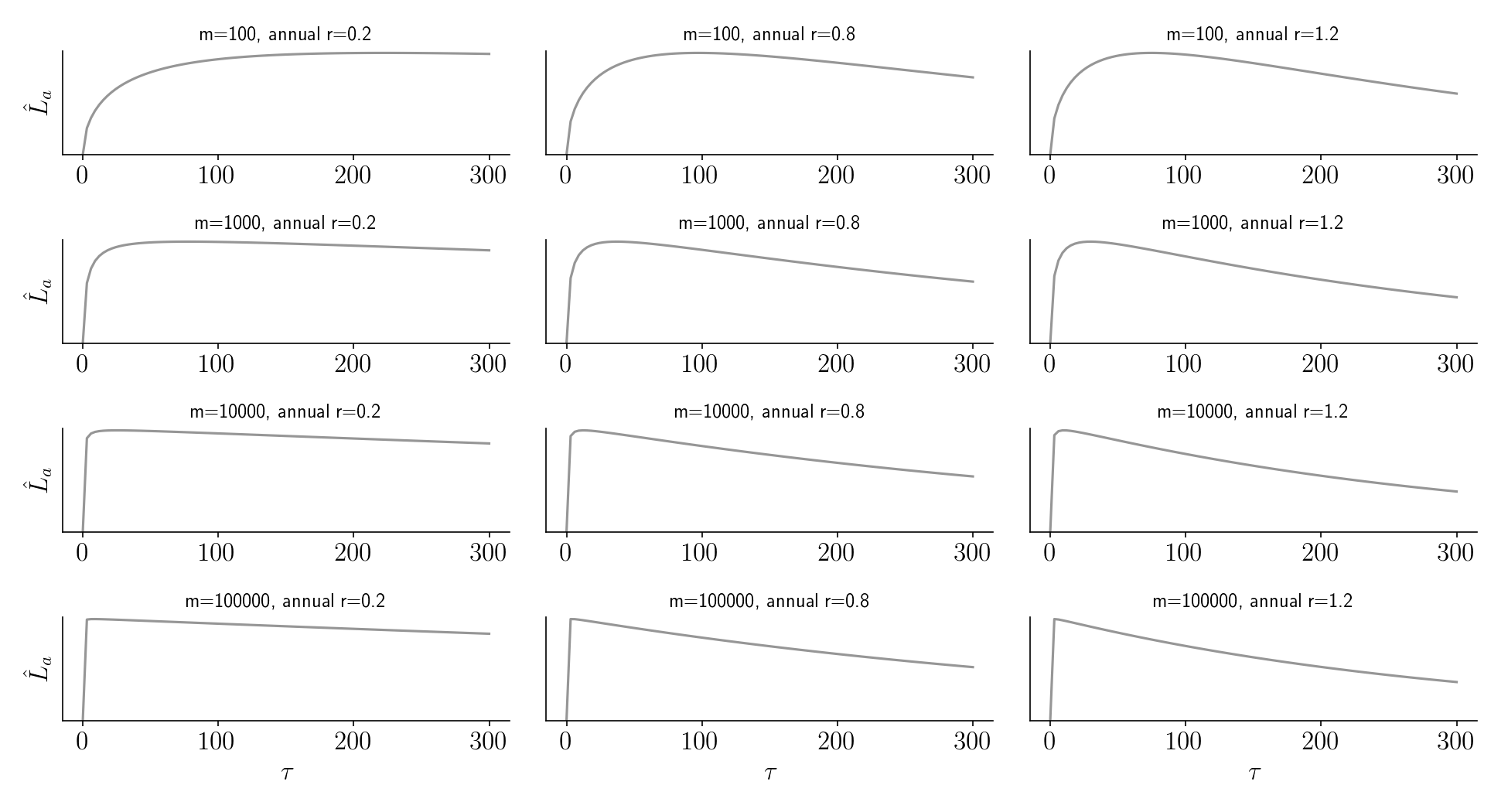Figure 5.

### Upcoming posts and Python notebook

You probably have a lot of questions about where this framework comes from and how it is justified. Part II and Part III dive more deeply into the math and visual intuition behind it. They also contain some example uses of the get_lift_via_closed_form and get_agg_lift_via_closed_form functions available in the accompanying Python Notebook.

# Variance after scaling and summing: One of the most useful facts from statistics

What do $R^2$, laboratory error analysis, ensemble learning, meta-analysis, and financial portfolio risk all have in common? The answer is that they all depend on a fundamental principle of statistics that is not as widely known as it should be. Once this principle is understood, a lot of stuff starts to make more sense.

Here’s a sneak peek at what the principle is.

Don’t worry if the formula doesn’t yet make sense! We’ll work our way up to it slowly, taking pit stops along the way at simpler formulas are that useful on their own. As we work through these principles, we’ll encounter lots of neat applications and explainers.

This post consists of three parts:

• Part 1: Sums of uncorrelated random variables: Applications to social science and laboratory error analysis
• Part 2: Weighted sums of uncorrelated random variables: Applications to machine learning and scientific meta-analysis
• Part 3: Correlated variables and Modern Portfolio Theory

## Part 1: Sums of uncorrelated random variables: Applications to social science and laboratory error analysis

Let’s start with some simplifying conditions and assume that we are dealing with uncorrelated random variables. If you take two of them and add them together, the variance of their sum will equal the sum of their variances. This is amazing!

To demonstrate this, I’ve written some Python code that generates three arrays, each of length 1 million. The first two arrays contain samples from two normal distributions with variances 9 and 16, respectively. The third array is the sum of the first two arrays. As shown in the simulation, its variance is 25, which is equal to the sum of the variances of the first two arrays (9 + 16).

from numpy.random import randn
import numpy as np
n = 1000000
x1 = np.sqrt(9) * randn(n) # 1M samples from normal distribution with variance=9
print(x1.var()) # 9
x2 = np.sqrt(16) * randn(n) # 1M samples from normal distribution with variance=16
print(x2.var()) # 16
xp = x1 + x2
print(xp.var()) # 25


This fact was first discovered in 1853 and is known as Bienaymé’s Formula. While the code example above shows the sum of two random variables, the formula can be extended to multiple random variables as follows:

If $X_p$ is a sum of uncorrelated random variables $X_1 .. X_n$, then the variance of $X_p$ will be $$\sigma_{p}^{2} = \sum{\sigma^2_i}$$ where each $X_i$ has variance $\sigma_i^2$.

What does the $p$ stand for in $X_p$? It stands for portfolio, which is just one of the many applications we’ll see later in this post.

### Why this is useful

Bienaymé’s result is surprising and unintuitive. But since it’s such a simple formula, it is worth committing to memory, especially because it sheds light on so many other principles. Let’s look at two of them.

#### Understanding $R^2$ and “variance explained”

Psychologists often talk about “within-group variance”, “between-group variance”, and “variance explained”. What do these terms mean?

Imagine a hypothetical study that measured the extraversion of 10 boys and 10 girls, where extraversion is measured on a 10-point scale (Figure 1. Orange bars). The boys have a mean extraversion of 4.4 and the girls have a mean extraversion 5.0. In addition, the overall variance of the data is 2.5. We can decompose this variance into two parts:

• Between-group variance: Create a 20-element array where every boy is assigned to the mean boy extraversion of 4.4, and every girl is assigned to the mean girl extraversion of 5.0. The variance of this array is 0.9. (Figure 1. Blue bars).
• Within-group variance: Create a 20-element array of the amount each child’s extraversion deviates from the mean value for their sex. Some of these values will be negative and some will be positive. The variance of this array is 1.6. (Figure 1. Pink bars).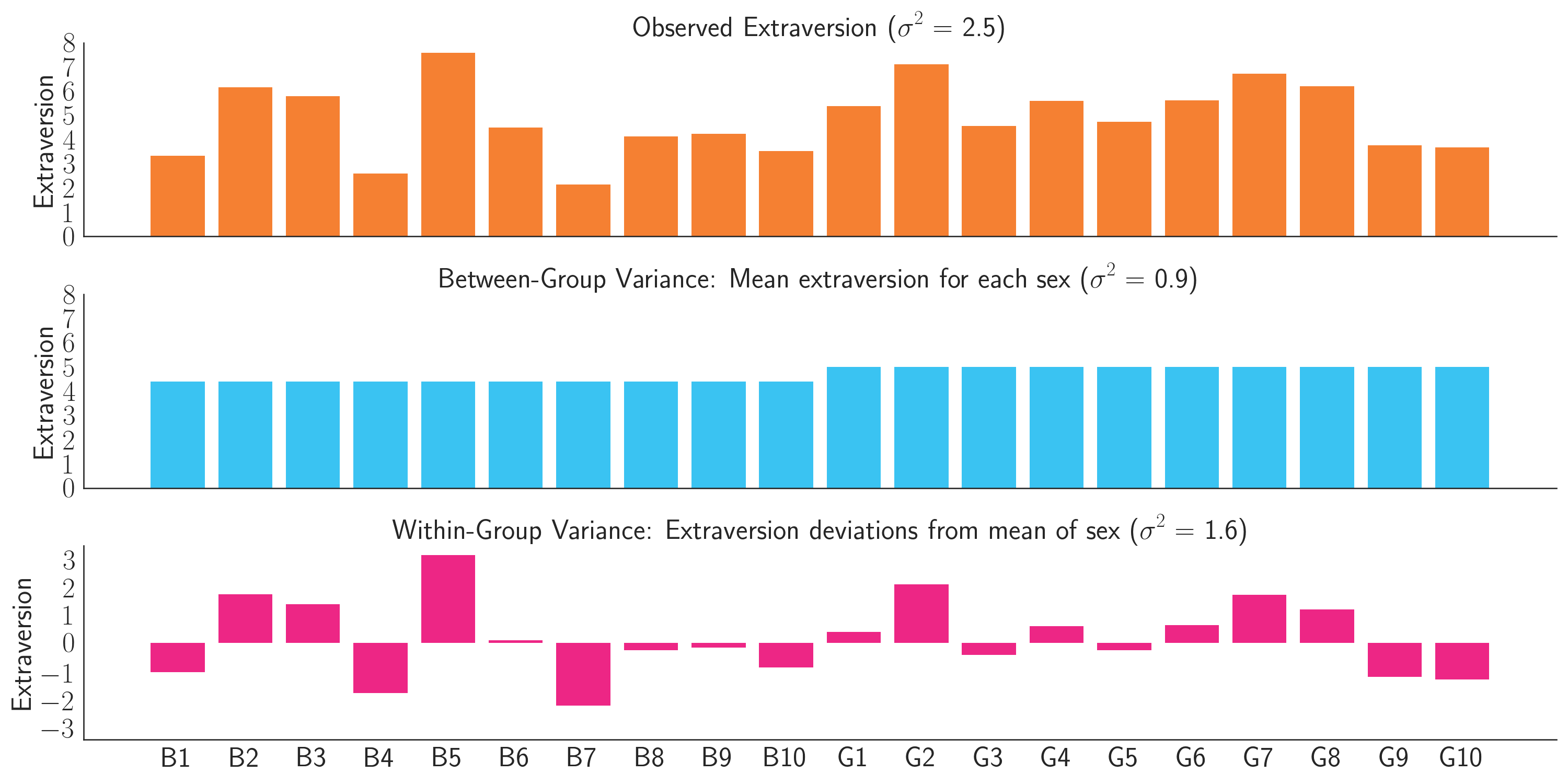Figure 1: Decomposition of extraversion scores (orange) into between-group variance (blue) and within-group variance (pink).

If you add these arrays together, the resulting array will represent the observed data (Figure 1. Orange bars). The variance of the observed array is 2.5, which is exactly what is predicted by Bienaymé’s Formula. It is the sum of the variances of the two component arrays (0.9 + 1.6). Psychologists might say that sex “explains” 0.9/2.5 = 36% of the extraversion variance. Equivalently, a model of extraversion that uses sex as the only predictor would have an $R^2$ of 0.36.

#### Error propagation in laboratories

If you ever took a physics lab or chemistry lab back in college, you may remember having to perform error analysis, in which you calculated how errors would propagate through one noisy measurement after another.

Physics textbooks often say that standard deviations add in “quadrature”, which just means that if you are trying to estimate some quantity that is the sum of two other measurements, and if each measurement has some error with standard deviation $\sigma_1$ and $\sigma_2$ respectively, the final standard deviation would be $\sigma_{p} = \sqrt{\sigma^2_1 + \sigma^2_2}$. I think it’s probably easier to just use variances, as in the Bienaymé Formula, with $\sigma^2_{p} = \sigma^2_1 + \sigma^2_2$.

For example, imagine you are trying to estimate the height of two boxes stacked on top of each other (Figure 2). One box has a height of 1 meter with variance $\sigma^2_1$ = 0.01, and the other has a height of 2 meters with variance $\sigma^2_2$ = 0.01. Let’s further assume, perhaps optimistically, that these errors are independent. That is, if the measurement of the first box is too high, it’s not any more likely that the measurement of the second box will also be too high. If we can make these assumptions, then the total height of the two boxes will be 3 meters with variance $\sigma^2_p$ = 0.02.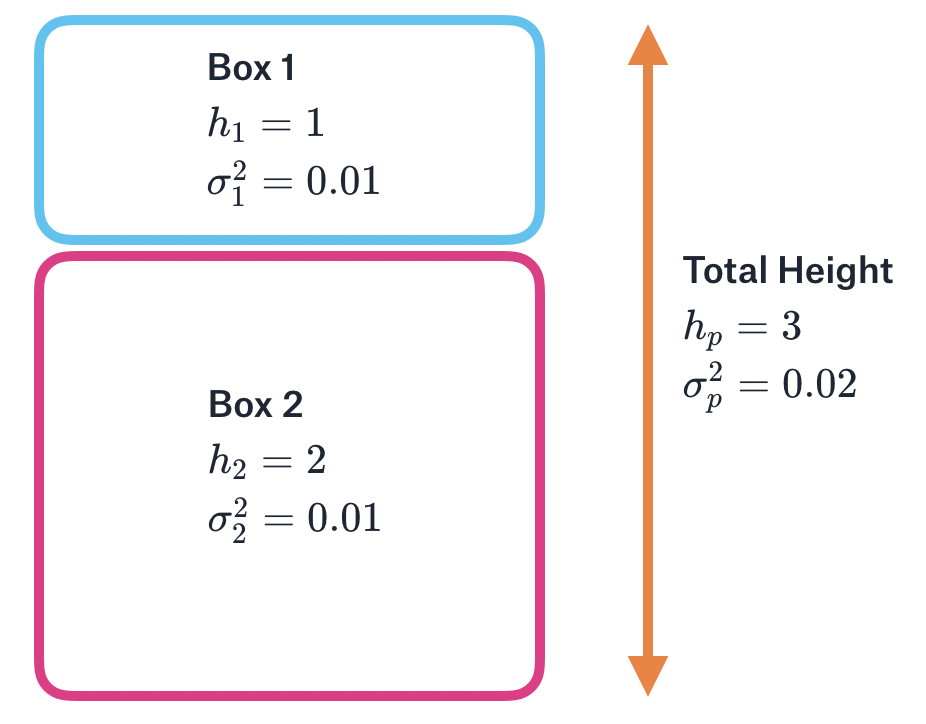Figure 2: Two boxes stacked on top of each other. The height of each box is measured with some variance (uncertainty). The total height is the sum of the individual heights, and the total variance (uncertainty) is the sum of the individual variances.

There is a key difference between the extraversion example and the stacked boxes example. In the extraversion example, we added two arrays that each had an observed sample variance. In the stacked boxes example, we added two scalar measurements, where the variance of these measurements refers to our measurement uncertainty. Since both cases have a meaningful concept of ‘variance’, the Bienaymé Formula applies to both.

## Part 2: Weighted sums of uncorrelated random variables: Applications to machine learning and scientific meta-analysis

Let’s now move on to the case of weighted sums of uncorrelated random variables. But before we get there, we first need to understand what happens to variance when a random variable is scaled.

If $X_p$ is defined as $X$ scaled by a factor of $w$, then the variance $X_p$ will be $$\sigma_{p}^{2} = w^2 \sigma^2$$ where $\sigma^2$ is the variance of $X$.

This means that if a random variable is scaled, the scale factor on the variance will change quadratically. Let’s see this in code.

from numpy.random import randn
import numpy as np
n = 1000000
baseline_var = 10
w = 0.7
x1 = np.sqrt(baseline_var) * randn(n) # Array of 1M samples from normal distribution with variance=10
print(x1.var()) # 10
xp = w * x1 # Scale this by w=0.7
print(w**2 * baseline_var) # 4.9 (predicted variance)
print(xp.var()) # 4.9 (empirical variance)


To gain some intuition for this rule, it’s helpful to think about outliers. We know that outliers have a huge effect on variance. That’s because the formula used to compute variance, $\sum{\frac{(x_i - \bar{x})^2}{n-1}}$, squares all the deviations, and so we get really big variances when we square large deviations. With that as background, let’s think about what happens if we scale our data by 2. The outliers will spread out twice as far, which means they will have even more than twice as much impact on the variance. Similarly, if we multiply our data by 0.5, we will squash the most “damaging” part of the outliers, and so we will reduce our variance by more than a factor of two.

While the above principle is pretty simple, things start to get interesting when you combine it with the Bienaymé Formula in Part I:

If $X_p$ is a weighted sum of uncorrelated random variables $X_1 ... X_n$, then the variance of $X_p$ will be $$\sigma_{p}^{2} = \sum{w^2_i \sigma^2_i}$$ where each $w_i$ is a weight on $X_i$, and each $X_i$ has its own variance $\sigma_i^2$.

The above formula shows what happens when you scale and then sum random variables. The final variance is the weighted sum of the original variances, where the weights are squares of the original weights. Let’s see how this can be applied to machine learning.

### An ensemble model with equal weights

Imagine that you have built two separate models to predict car prices. While the models are unbiased, they have variance in their errors. That is, sometimes a model prediction will be too high, and sometimes a model prediction will be too low. Model 1 has a mean squared error (MSE) of \$1,000 and Model 2 has an MSE of \$2,000.

A valuable insight from machine learning is that you can often create a better model by simply averaging the predictions of other models. Let’s demonstrate this with simulations below.

from numpy.random import randn
import numpy as np
n = 1000000
actual = 20000 + 5000 * randn(n)
errors1 = np.sqrt(1000) * randn(n)
print(errors1.var()) # 1000
errors2 = np.sqrt(2000) * randn(n)
print(errors2.var()) # 2000

# Note that this section could be replaced with
# errors_ensemble = 0.5 * errors1 + 0.5 * errors2
preds1 = actual + errors1
preds2 = actual + errors2
preds_ensemble = 0.5 * preds1 + 0.5 * preds2
errors_ensemble = preds_ensemble - actual

print(errors_ensemble.var()) # 750. Lower than variance of component models!


As shown in the code above, even though a good model (Model 1) was averaged with an inferior model (Model 2), the resulting Ensemble model’s MSE of \$750 is better than either of the models individually. The benefits of ensembling follow directly from the weighted sum formula we saw above, $\sigma_{p}^{2} = \sum{w^2_i \sigma^2_i}$. To understand why, it’s helpful to think of models not as generating predictions, but rather as generating errors. Since averaging the predictions of a model corresponds to averaging the errors of the model, we can treat each model’s array of errors as samples of a random variable whose variance can be plugged in to the formula. Assuming the models are unbiased (i.e. the errors average to about zero), the formula tells us the expected MSE of the ensemble predictions. In the example above, the MSE would be which is exactly what we observed in the simulations. (For a totally different intuition of why ensembling works, see this blog post that I co-wrote for my company, Opendoor.) ### An ensemble model with Inverse Variance Weighting In the example above, we obtained good results by using an equally-weighted average of the two models. But can we do better? Yes we can! Since Model 1 was better than Model 2, we should probably put more weight on Model 1. But of course we shouldn’t put all our weight on it, because then we would throw away the demonstrably useful information from Model 2. The optimal weight must be somewhere in between 50% and 100%. An effective way to find the optimal weight is to build another model on top of these models. However, if you can make certain assumptions (unbiased and uncorrelated errors), there’s an even simpler approach that is great for back-of-the envelope calculations and great for understanding the principles behind ensembling. To find the optimal weights (assuming unbiased and uncorrelated errors), we need to minimize the variance of the ensemble errors $\sigma_{p}^{2} = \sum{w^2_i \sigma^2_i}$ with the constraint that $\sum{w_i} = 1$. It turns out that the variance-minimizing weight for a model should be proportional to the inverse of its variance. When we apply this method, we obtain optimal weights of $w_1$ = 0.67 and $w_2$ = 0.33. These weights give us an ensemble error variance of which is significantly better than the$750 variance we were getting with equal weighting.

This method is called Inverse Variance Weighting, and allows you to assign the right amount of weight to each model, depending on its error.

Inverse Variance Weighting is not just useful as a way to understand Machine Learning ensembles. It is also one of the core principles in scientific meta-analysis, which is popular in medicine and the social sciences. When multiple scientific studies attempt to estimate some quantity, and each study has a different sample size (and hence variance of their estimate), a meta-analysis should weight the high sample size studies more. Inverse Variance Weighting is used to determine those weights.

## Part 3: Correlated variables and Modern Portfolio Theory

Let’s imagine we now have three unbiased models with the following MSEs:

• Model 1: MSE = 1000
• Model 2: MSE = 1000
• Model 3: MSE = 2000

By Inverse Variance Weighting, we should assign more weight to the first two models, with $w_1=0.4, w_2=0.4, w_3=0.2$.

But what happens if Model 1 and Model 2 have correlated errors? For example, whenever Model 2’s predictions are too high, Model 3’s predictions tend to also be too high. In that case, maybe we don’t want to give so much weight to Models 1 and 2, since they provide somewhat redundant information. Instead we might want to diversify our ensemble by increasing the weight on Model 3, since it provides new independent information.

To determine how much weight to put on each model, we first need to determine how much total variance there will be if the errors are correlated. To do this, we need to borrow a formula from the financial literature, which extends the formulas we’ve worked with before. This is the formula we’ve been waiting for.

If $X_p$ is a weighted sum of (correlated or uncorrelated) random variables $X_1 ... X_n$, then the variance of $X_p$ will be $$\sigma_{p}^{2} = \sum\limits_{i} \sum\limits_{j} w_i w_j \sigma_i \sigma_j \rho_{ij}$$ where each $w_i$ and $w_j$ are weights assigned to $X_i$ and $X_j$, where each $X_i$ and $X_j$ have standard deviations $\sigma_i$ and $\sigma_j$, and where the correlation between $X_i$ and $X_j$ is $\rho_{ij}$.

There’s a lot to unpack here, so let’s take this step by step.

• $\sigma_i \sigma_j \rho_{ij}$ is a scalar quantity representing the covariance between $X_i$ and $X_j$.
• If none of the variables are correlated with each other, then all the cases where $i \neq j$ will go to zero, and the formula reduces to $\sigma_{p}^{2} = \sum{w^2_i \sigma^2_i}$, which we have seen before.
• The more that two variables $X_i$ and $X_j$ are correlated, the more the total variance $\sigma_{p}^{2}$ increases.
• If two variables $X_i$ and $X_j$ are anti-correlated, then the total variance decreases, since $\sigma_i \sigma_j \rho_{ij}$ is negative.
• This formula can be rewritten in more compact notation as $\sigma_{p}^{2} = \vec{w}^T\Sigma \vec{w}$, where $\vec{w}$ is the weight vector, and $\Sigma$ is the covariance matrix (not a summation sign!)

If you skimmed the bullet points above, go back and re-read them! They are super important.

To find the set of weights that minimize variance in the errors, you must minimize the above formula, with the constraint that $\sum{w_i} = 1$. One way to do this is to use a numerical optimization method. In practice, however, it is more common to just find weights by building another model on top of the base models

Regardless of how the weights are found, it will usually be the case that if Models 1 and 2 are correlated, the optimal weights will reduce redundancy and put lower weight on these models than simple Inverse Variance Weighting would suggest.

### Applications to financial portfolios

The formula above was discovered by economist Harry Markowitz in his Modern Portfolio Theory, which describes how an investor can optimally trade off between expected returns and expected risk, often measured as variance. In particular, the theory shows how to maximize expected return given a fixed variance, or minimize variance given a fixed expected return. We’ll focus on the latter.

Imagine you have three stocks to put in your portfolio. You plan to sell them at time $T$, at which point you expect that Stock 1 will have gone up by 5%, with some uncertainty. You can describe your uncertainty as variance, and in the case of Stock 1, let’s say $\sigma_1^2$ = 1. This stock, as well Stocks 2 and 3, are summarized in the table below:

Stock ID Expected Return Expected Risk ($\sigma^2$)
1 5.0 1.0
2 5.0 1.0
3 5.0 2.0

This financial example should remind you of ensembling in machine learning. In the case of ensembling, we wanted to minimize variance of the weighted sum of error arrays. In the case of financial portfolios, we want to minimize the variance of the weighted sum of scalar financial returns.

As before, if there are no correlations between the expected returns (i.e. if Stock 1 exceeding 5% return does not imply that Stock 2 or Stock 3 will exceed 5% return), then the total variance in the portfolio will be $\sigma_{p}^{2} = \sum{w^2_i \sigma^2_i}$ and we can use Inverse Variance Weighting to obtain weights $w_1=0.4, w_2=0.4, w_3=0.2$.

However, sometimes stocks have correlated expected returns. For example, if two of the stocks are in oil companies, then one stock exceeding 5% implies the other is also likely to exceed 5%. When this happens, the total variance becomes

as we saw before in the ensemble example. Since this includes an additional positive term for $w_1 w_2 \sigma_1 \sigma_2 \rho_{1,2}$, the expected variance is higher than in the uncorrelated case, assuming the correlations are positive. To reduce this variance, we should put less weight on Stocks 1 and 2 than we would otherwise.

While the example above focused on minimizing the variance of a financial portfolio, you might also be interested in having a portfolio with high return. Modern Portfolio Theory describes how a portfolio can reach any abitrary point on the efficient frontier of variance and return, but that’s outside the scope of this blog post. And as you might expect, financial markets can be more complicated than Modern Portfolio Theory suggests, but that’s also outside scope.

## Summary

That was a long post, but I hope that the principles described have been informative. It may be helpful to summarize them in backwards order, starting with the most general principle.

If $X_p$ is a weighted sum of (correlated or uncorrelated) random variables $X_1 ... X_n$, then the variance of $X_p$ will be $$\sigma_{p}^{2} = \sum\limits_{i} \sum\limits_{j} w_i w_j \sigma_i \sigma_j \rho_{ij}$$ where each $w_i$ and $w_j$ are weights assigned to $X_i$ and $X_j$, where each $X_i$ and $X_j$ have standard deviations $\sigma_i$ and $\sigma_j$, and where the correlation between $X_i$ and $X_j$ is $\rho_{ij}$. The term $\sigma_i \sigma_j \rho_{ij}$ is a scalar quantity representing the covariance between $X_i$ and $X_j$.

If none of the variables are correlated, then all the cases where $i \neq j$ go to zero, and the formula reduces to $$\sigma_{p}^{2} = \sum{w^2_i \sigma^2_i}$$ And finally, if we are computing a simple sum of random variables where all the weights are 1, then the formula reduces to $$\sigma_{p}^{2} = \sum{\sigma^2_i}$$

# Using your ears and head to escape the Cone Of Confusion

One of coolest things I ever learned about sensory physiology is how the auditory system is able to locate sounds. To determine whether sound is coming from the right or left, the brain uses inter-ear differences in amplitude and timing. As shown in the figure below, if the sound is louder in the right ear compared to the left ear, it’s probably coming from the right side. The smaller that difference is, the closer the sound is to the midline (i.e the vertical plane going from your front to your back). Similarly, if the sound arrives at your right ear before the left ear, it’s probably coming from the right. The smaller the timing difference, the closer it is to the midline. There’s a fascinating literature on the neural mechanisms behind this.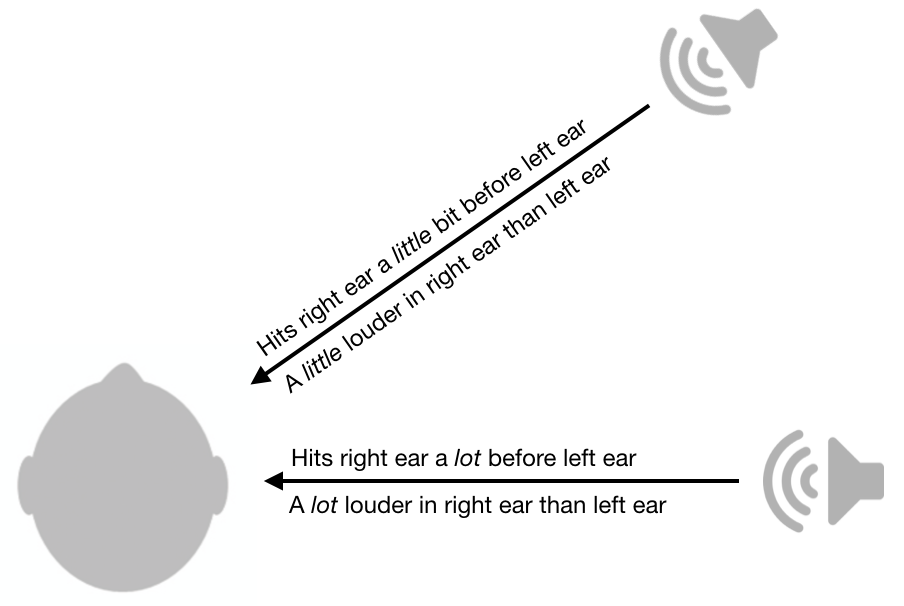Inter-ear loudness and timing differences are pretty useful, but unfortunately they still leave a lot of ambiguity. For example, a sound from your front right will have the exact same loudness differences and timing differences as a sound from your back right.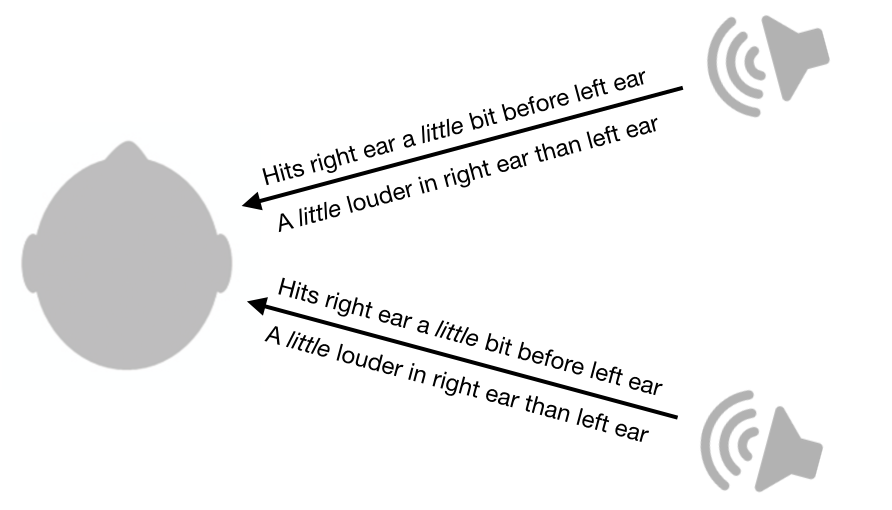Not only does this system leave ambiguities between front and back, it also leaves ambiguities between top and down. In fact, there is an entire cone of confusion that cannot be disambiguated by this system. Sound from all points along the surface of the cone will have the same inter-ear loudness differences and timing differences.While this system leaves a cone of confusion, humans are still able to determine the location of sounds from different points on the cone, at least to some extent. How are we able to do this?

Amazingly, we are able to do this because of the shape of our ears and heads. When sound passes through our ears and head, certain frequencies are attenuated more than others. Critically, the attenuation pattern is highly dependent on sound direction.

This location-dependent attenuation pattern is called a Head-related transfer function (HRTF) and in theory this could be used to disambiguate locations along the cone of confusion. An example of someone’s HRTF is shown below, with frequency on the horizontal axis and polar angle on the vertical axis. Hotter colors represent less attenuation (i.e. more power). If your head and ears gave you this HRTF, you might decide a sound is coming from the front if it has more high frequency power than you’d expect.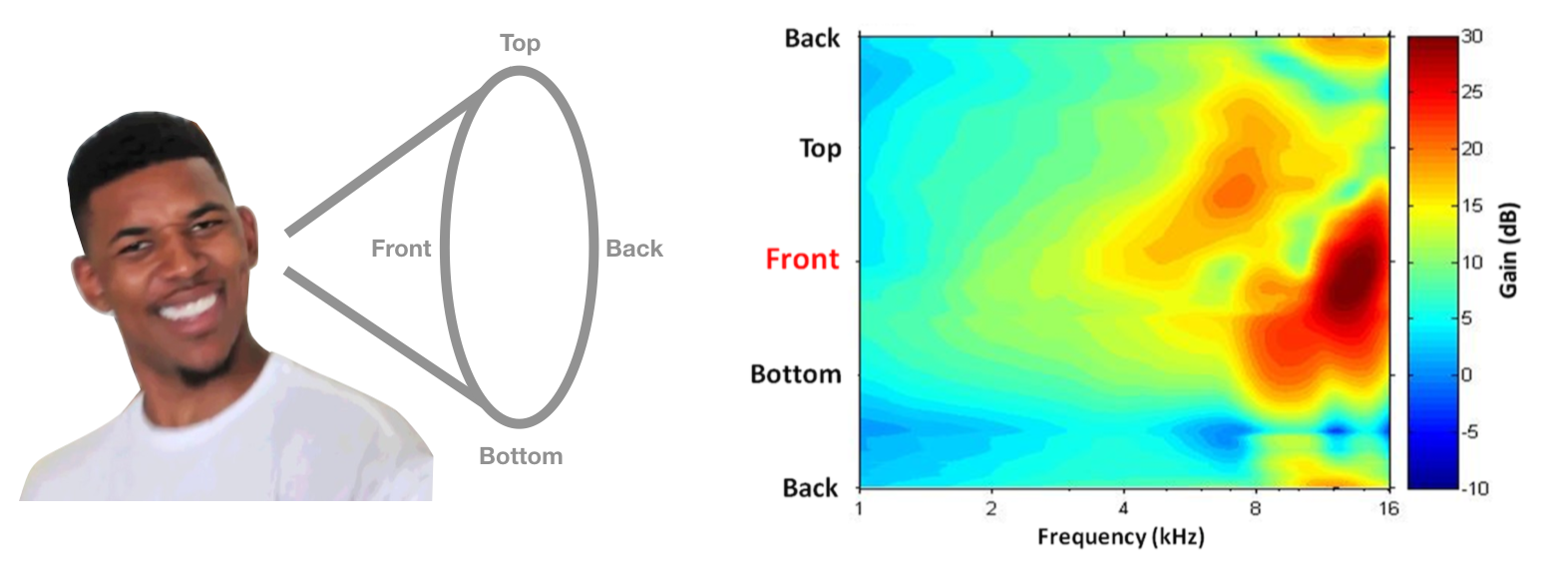HRTF image from Simon Carlile's Psychoacoustics chapter in The Sonification Handbook.

This system sounds good in theory, but do we actually use these cues in practice? In 1988, Frederic Wightman and Doris Kistler performed an ingenious set of experiments (1, 2) to show that that people really do use HRTFs to infer location. First, they measured the HRTF of each participant by putting a small microphone in their ears and playing sounds from different locations. Next they created a digital filter for each location and each participant. That is to say, these filters implemented each participant’s HRTF. Finally, they placed headphones on the listeners and played sounds to them, each time passing the sound through one of the digital filters. Amazingly, participants were able to correctly guess the “location” of the sound, depending on which filter was used, even though the sound was coming from headphones. They were also much better at sound localization when using their own HRTF, rather than someone else’s HRTF.

Further evidence for this hypothesis comes from Hofman et al., 1998, who showed that by using putty to reshape people’s ears, they were able to change the HRTFs and thus disrupt sound localization. Interestingly, people were able to quickly relearn how to localize sound with their new HRTFs.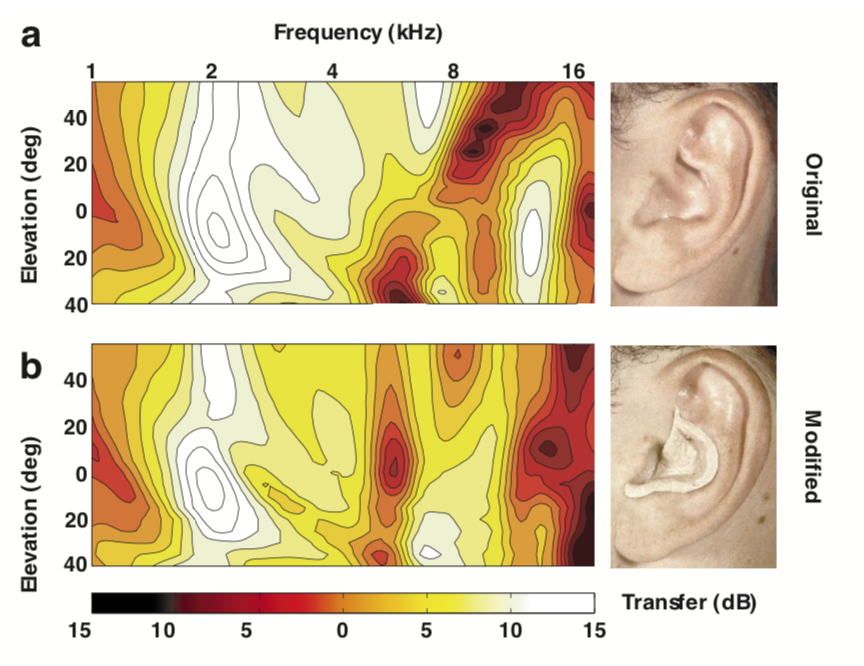Image from Hofman et al., 1998.

A final fun fact: to improve the sound localization of humanoid robots, researchers in Japan attached artificial ears to the robot heads and implemented some sophisticated algorithms to infer sound location. Here are some pictures of the robots.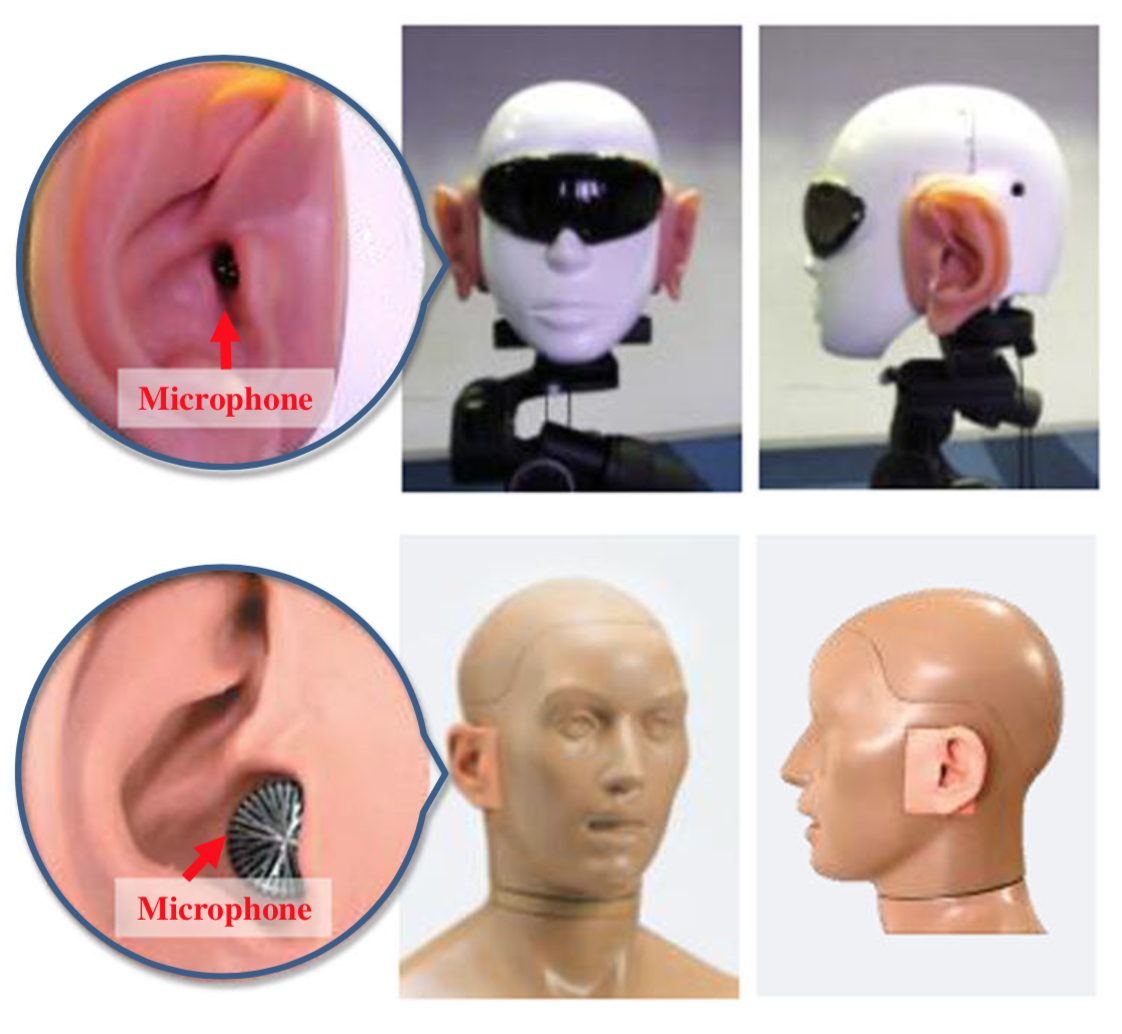Their paper is kind of ridiculous and has some questionable justifications for not just using microphones in multiple locations, but I thought it was fun to see these principles being applied.